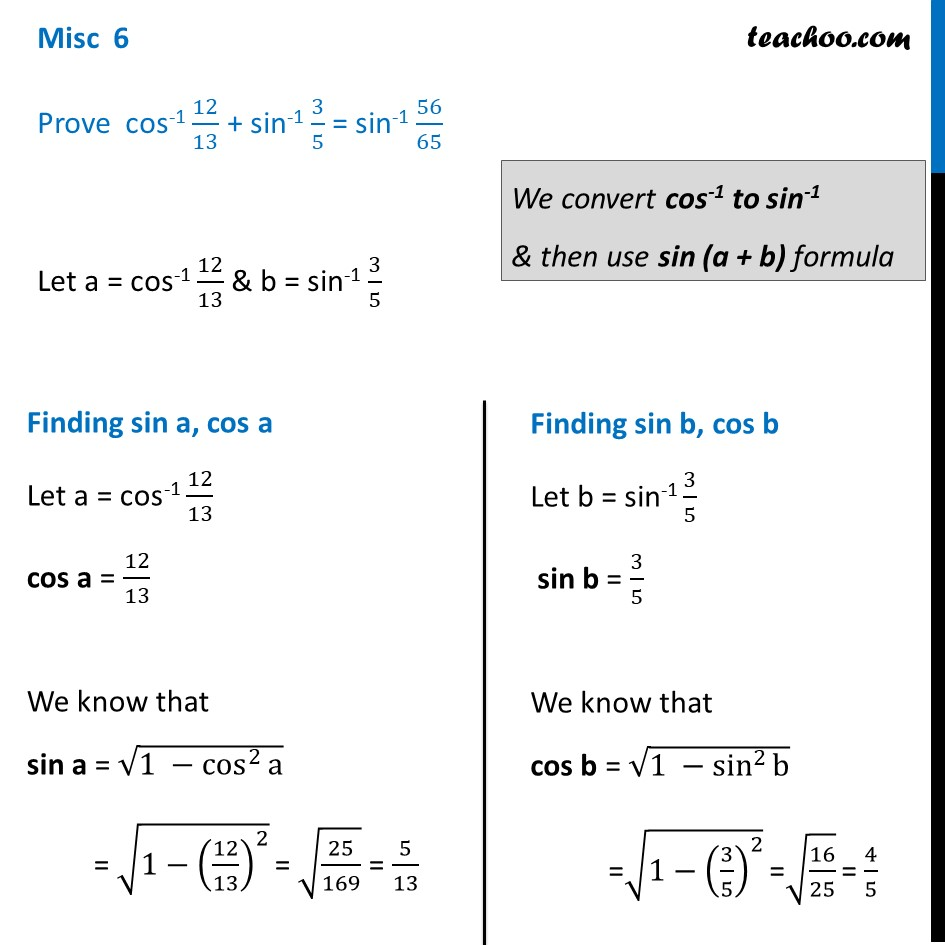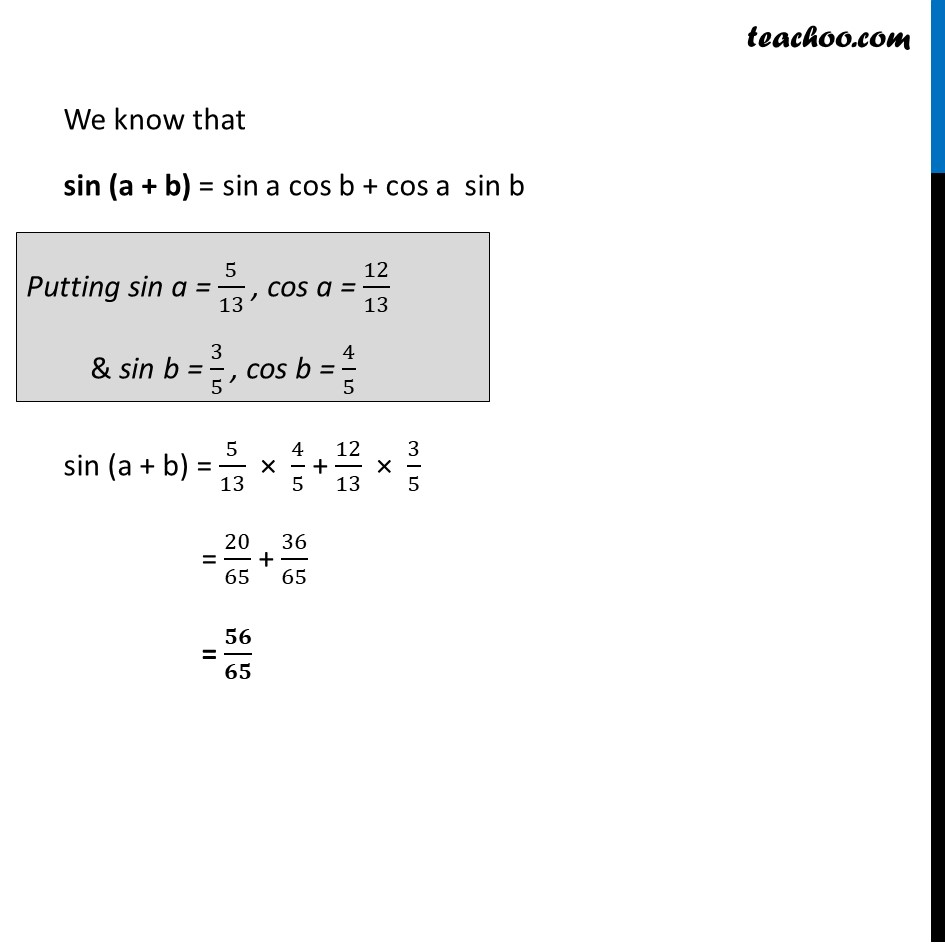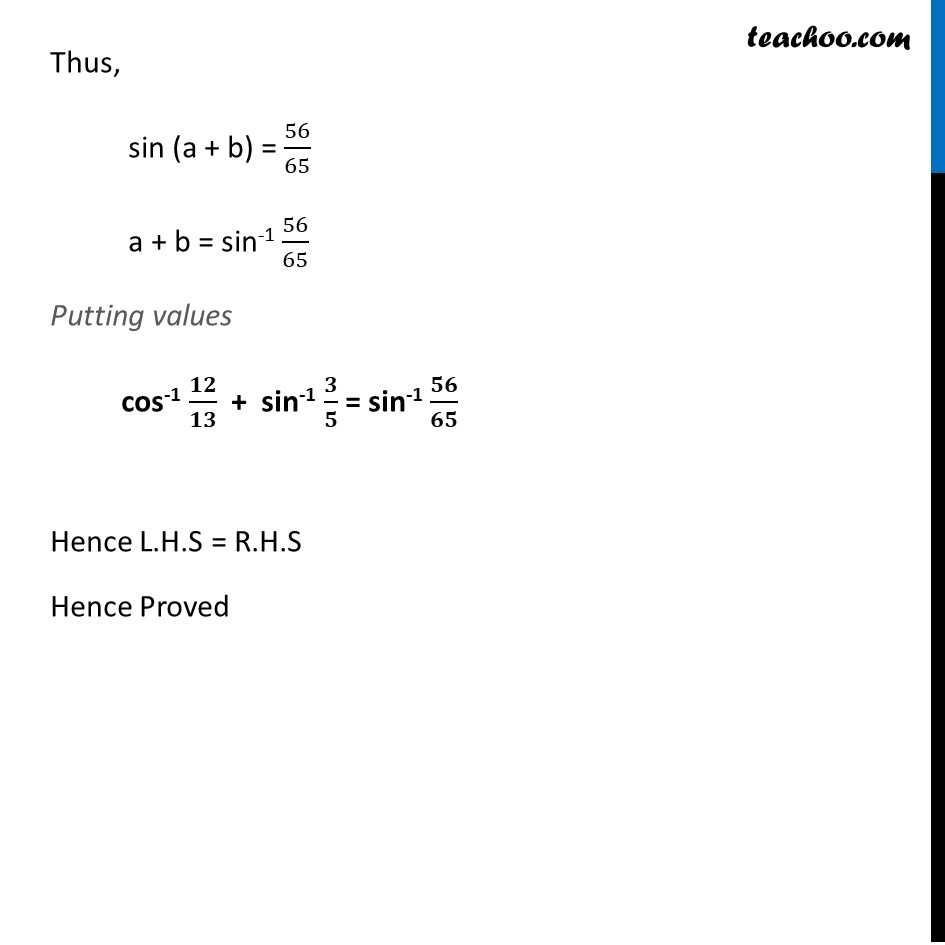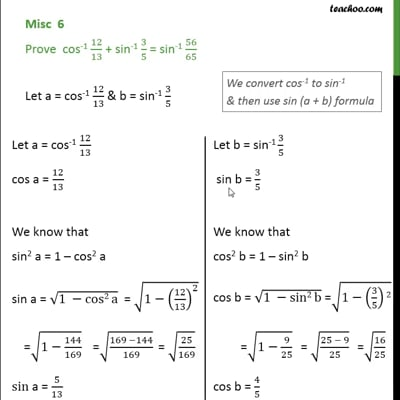Miscellaneous

Chapter 2 Class 12 Inverse Trigonometric Functions
Serial order wiseThis video is only available for Teachoo black users

Solve all your doubts with Teachoo Black (new monthly pack available now!)

### Transcript

Misc 6 Prove cos-1 12/13 + sin-1 3/5 = sin-1 56/65 Let a = cos-1 12/13 & b = sin-1 3/5 Finding sin a, cos a Let a = cos-1 12/13 cos a = 12/13 We know that sin a = √(1 −cos^2⁡a ) = √(1−(12/13)^2 ) "=" √(25/169) = 5/13 We convert cos-1 to sin-1 & then use sin (a + b) formula Finding sin b, cos b Let b = sin-1 3/5 sin b = 3/5 We know that cos b = √(1 −sin^2⁡b ) =√(1−(3/5)^2 ) "=" √(16/25) "=" 4/5 We know that sin (a + b) = sin a cos b + cos a sin b sin (a + b) = 5/13 × 4/5 + 12/13 × 3/5 = 20/65 + 36/65 = 𝟓𝟔/𝟔𝟓 Putting sin a = 5/13 , cos a = 12/13 & sin b = 3/5 , cos b = 4/5 Thus, sin (a + b) = 56/65 a + b = sin-1 56/65 Putting values cos-1 𝟏𝟐/𝟏𝟑 + sin-1 𝟑/𝟓 = sin-1 𝟓𝟔/𝟔𝟓 Hence L.H.S = R.H.S Hence Proved